# Superconductor: models with dependence on B

## Power law with dependences on B

The critical current density Jc and the exponent n are the quantities that depend on the magnetic flux density B.

The general form of the previous function is thus the following one: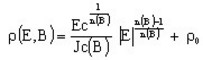(3)

where:

• Ec is the critical electric field [V/m]
• Jc(B) is the dependence on B of the critical current density (see below)
• n(B) is the dependence on B of the exponent (see below)
• ρ0 is an additional resistivity [Ω.m]

The dependence on B of the quantities Jc and n can be expressed using different models as that is presented in the tables below.

Isotropic dependence, Kim-Anderson model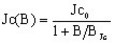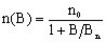2 additional parameters: BJc and Bn
Anisotropic dependence, Kim-Anderson model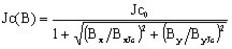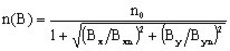4 additional parameters: BxJc ByJc and Bxn Byn
Isotropic dependence, exponential function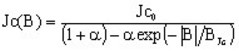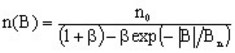4 additional parameters: α β BJc and Bn
Anisotropic dependence, exponential function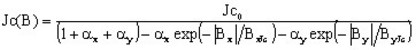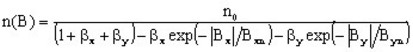8 additional parameters: αx αy βx βy BxJc ByJc and Bxn Byn
 Isotropic dependence, polynomial function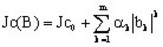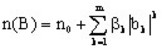2 m additional parameters: αk βk